# Spring Mass System

## What is Spring Mass System?

Consider a spring with mass m with spring constant k, in a closed environment spring demonstrates a simple harmonic motion.

T = 2π √m/k

From the above equation, it is clear that the period of oscillation is free from both gravitational acceleration and amplitude. Also, a constant force cannot alter the period of oscillation.

## Parallel Combination of Springs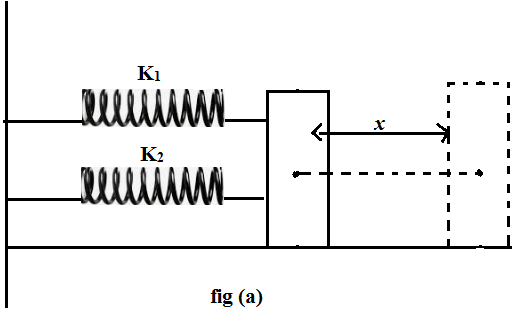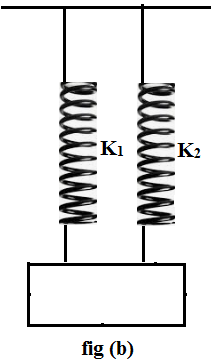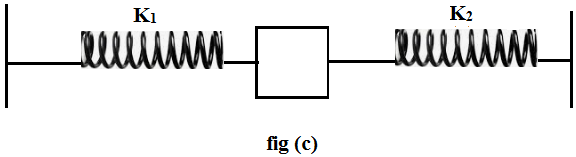Fig (a), (b) and (c) – are the parallel combination of springs.

Displacement on each spring = is same

But restoring force = is different

$F={{F}_{1}}+{{F}_{2}}$

$-{{k}_{p}}x=-{{k}_{1}}x-{{k}_{2}}x$

$-x{{k}_{p}}=-x\left( {{k}_{1}}+{{k}_{2}} \right)$

${{k}_{p}}={{k}_{1}}+{{k}_{2}}$

### Time Period in Parallel Combination

$T=\frac{2\pi }{\omega }=2\pi \sqrt{\frac{m}{{{k}_{p}}}}=2\pi \sqrt{\frac{m}{{{k}_{1}}+{{k}_{2}}}}$

## Springs in Series Combination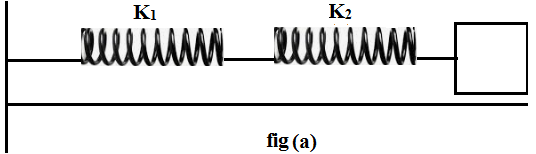Force on each string is same but displacement on each string is different

$x={{x}_{1}}+{{x}_{2}}$ ${{F}_{1}}=-{{k}_{1}}{{x}_{1}}$

$T=2\pi \sqrt{\frac{L}{{{g}_{eff}}}}=2\pi \sqrt{\frac{L}{g+a}}$ ${{F}_{2}}=-{{k}_{2}}{{x}_{2}}$

$\frac{1}{{{k}_{s}}}=\frac{1}{{{k}_{1}}}+\frac{1}{{{k}_{2}}}$ $\frac{F}{{{k}_{1}}}={{x}_{1}}$

${{k}_{s}}=\frac{{{k}_{1}}{{k}_{2}}}{{{k}_{1}}+{{k}_{2}}}$ $\frac{F}{{{k}_{2}}}={{x}_{2}}$

### Time Period in Series Combination

$T=2\pi \sqrt{\frac{m}{{{k}_{s}}}}=2\pi \sqrt{\frac{m\left( {{k}_{1}}+{{k}_{2}} \right)}{{{k}_{1}}{{k}_{2}}}}$

### Spring Constant

$K=\frac{YA}{L}$ Y = youngs modulus of elasticity

From Hooke’s law

$Y=\frac{Stress}{Strain}=\frac{\frac{F}{A}}{\frac{DL}{L}}$ $\frac{YDL}{L}=\frac{F}{A}$ $F=\frac{YA}{L}\left( DL \right)$ $\left[ Since, \;K=\frac{YA}{L} \right]$

$F=K\,x$

$K=\frac{YA}{L}$

$K\propto \frac{1}{L}$

If a spring of spring constant (K) and length (L) cutted into $\frac{L}{2}$ size two pieces, then magnitude of spring constant of the new pieces will be?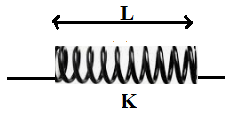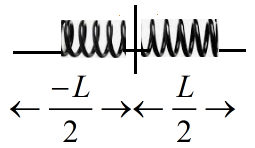$K\propto \frac{1}{L}\Rightarrow$ then K becomes = 2K for the new pieces.

### How to Find the Time period of a Spring Mass System?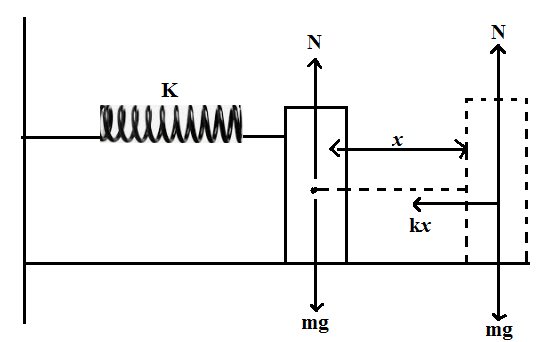Steps:

1. Find the mean position of the SHM (point at which Fnet = 0) in horizontal spring-mass system

The natural length of the spring = is the position of equilibrium point.

2. Displace the object by a small distance (x) from its equilibrium position (or) mean position other than mean position, restoring force will act on the body

$\overrightarrow{{{F}_{net}}}=-k\overrightarrow{x}$

$\overrightarrow{a}=\frac{-k}{m}\overrightarrow{x}$

3. Acceleration of the particle is calculated and the calculated value of $\overrightarrow{a}$.

$\overrightarrow{a}\propto -\overrightarrow{x}$ then only it is SHM

Then equate the $\overrightarrow{a}=-{{\omega }^{2}}\overrightarrow{x}$ $\frac{-k}{m}\overrightarrow{x}=-{{\omega }^{2}}\overrightarrow{x}$

$\omega =\sqrt{\frac{k}{m}}$

4. Substitute ω value in standard time period expression of SHM

$T=\frac{2\pi }{\omega }=\frac{2\pi }{\sqrt{\frac{k}{m}}}=2\pi \sqrt{\frac{m}{k}}$ $T=2\sqrt{\frac{Inertia}{Force\,constant}}$

## Problems on Spring Mass System

Q.1: A particle is executing linear SHM what are its velocity and displacement when its acceleration is half the maximum possible?

Solution:

$\overrightarrow{a}=-A{{\omega }^{2}}\sin \left( \omega t+\phi \right)$

$\overrightarrow{{{a}_{\max }}}=-A{{\omega }^{2}}$

$\frac{{{a}_{\max }}}{2}=-\frac{A{{\omega }^{2}}}{2}=-A{{\omega }^{2}}\sin \left( \frac{\pi }{6} \right)$

Phase $\left( \omega t+\phi \right)=\frac{\pi }{6}$

$v=A\omega \cos \left( \frac{\pi }{6} \right)=A\omega \frac{\sqrt{3}}{2}$

$x=A\sin \left( \frac{\pi }{6} \right)=\frac{A}{2}$

$\left( v=A\omega \frac{\sqrt{3}}{2},\,and\,\,x=\frac{A}{2} \right)$

Q.2: A particle executing linear SHM has speeds v1 and v2 at distances y1 and y2 from the equilibrium position. What is the frequency of the oscillation of the particle?

Solution:

$v=\omega \sqrt{{{A}^{2}}-{{y}^{2}}}$

${{v}^{2}}={{\omega }^{2}}\left( {{A}^{2}}-{{y}^{2}} \right)$

$\frac{{{v}^{2}}}{{{\omega }^{2}}}=\left( {{A}^{2}}-{{y}^{2}} \right)$

$\frac{{{v}^{2}}}{{{\omega }^{2}}}+{{y}^{2}}={{A}^{2}}$ … (1)

${{A}^{2}}=\frac{v_{1}^{2}}{{{\omega }^{2}}}+y_{1}^{2}=\frac{v_{2}^{2}}{{{\omega }^{2}}}+y_{2}^{2}$

$\frac{v_{1}^{2}-v_{2}^{2}}{{{\omega }^{2}}}=y_{2}^{2}-y_{1}^{2}$

${{\omega }^{2}}=\frac{v_{1}^{2}-v_{2}^{2}}{y_{2}^{2}-y_{1}^{2}}$

$\omega =2\pi f$

$f=\frac{\omega }{2\pi }=\frac{1}{2\pi }{{\left[ \frac{v_{1}^{2}-v_{2}^{2}}{y_{2}^{2}-y_{1}^{2}} \right]}^{\frac{1}{2}}}$

Q.3: A particle is executing SHM of amplitude A.

(a) What fraction of the total energy is kinetic when displacement is quarter of the amplitude?

(b) At what displacement is the energy are half kinetic and half potential?

(a) $90%,\,\,\frac{A}{\sqrt{2}}$

(b) $94%,\,\,\frac{A}{\sqrt{3}}$

(c) $9%,\,\,\frac{A}{\sqrt{4}}$

(d) $93%,\,\,\frac{A}{\sqrt{2}}$

Solution:

$KE=\frac{1}{2}m{{\omega }^{2}}\left( {{A}^{2}}-{{y}^{2}} \right)$

$PE=\frac{1}{2}m{{\omega }^{2}}{{y}^{2}}$

$E=\frac{1}{2}m{{\omega }^{2}}{{A}^{2}}$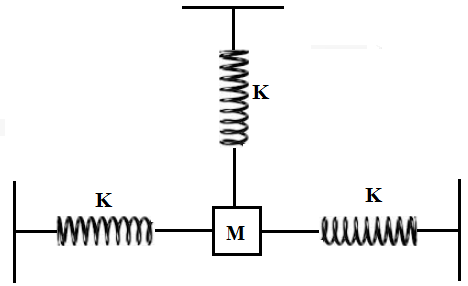(a) at $y=\frac{A}{4},$ KE becomes

$KE=\frac{1}{2}m{{\omega }^{2}}\left( {{A}^{2}}-{{\left( \frac{A}{4} \right)}^{2}} \right)$

= $\frac{15}{16}\frac{1}{2}m{{\omega }^{2}}{{A}^{2}}$

= 93% of total energy is KE

(b) KE = PE

$\frac{1}{2}m{{\omega }^{2}}\left( {{A}^{2}}-{{y}^{2}} \right)=\frac{1}{2}m{{\omega }^{2}}{{y}^{2}}$ $y=\frac{A}{\sqrt{2}}$

Q.4: Three springs each of force constant k are connected at equal angles with respect to each other to a common mass. If the mass is pulled by anyone of the spring then the time period of its oscillation?

(a) $2\pi \sqrt{\frac{M}{K}}$

(b) $2\pi \sqrt{\frac{M}{2K}}$

(c) $2\pi \sqrt{\frac{2M}{3K}}$

(d) $2\pi \sqrt{\frac{2M}{K}}$

Solution:

It is pulled by an upper spring each are making equal angles.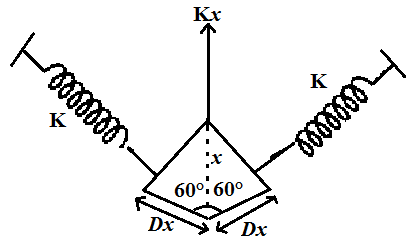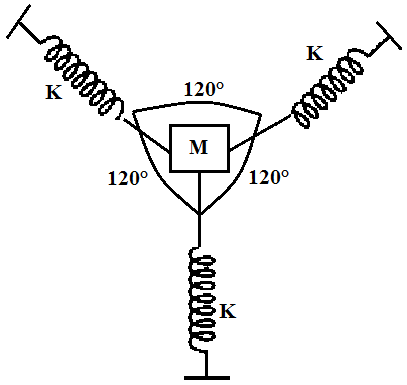$\cos 60{}^\circ =\frac{\Delta x}{x}$

$x\cos 60{}^\circ =\Delta \,x$

$\frac{x}{2}=\Delta \,x$

Fnet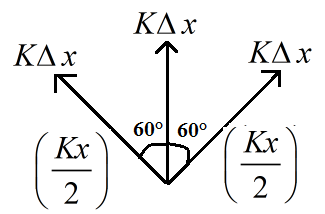${{F}_{net}}=Kx+2\frac{Kx}{2}\cos 60{}^\circ$

= $Kx+\frac{Kx}{2}=\frac{3Kx}{2}$

${{K}_{eqn}}x=\frac{3Kx}{2}$

${{K}_{eqn}}=\frac{3K}{2}$

$T=2\pi \sqrt{\frac{M}{K}}=2\pi \sqrt{\frac{2M}{3K}}$

Q.5: A particle of mass 0.2 kg is executing SHM of amplitude 0.2 m. When the particle passes through the mean position. It mechanical energy is $4\times {{10}^{-3}}J$ find the equation of motion of the particle if the initial phase of oscillation is 60°.

(a) $0.1\sin \left( 2t+\frac{\pi }{4} \right)$

(b) $0.2\sin \left( \frac{1}{2}t+\frac{\pi }{3} \right)$

(c) $0.2\sin \left( t+\frac{\pi }{3} \right)$

(d) $0.1\cos \left( 2t+\frac{\pi }{4} \right)$

Solution:

Equation of motion of particle is

$y=A\sin \left( \omega t+\phi \right)$

A = 0.2 m, $\omega =?,\,\,\phi =60{}^\circ ,\,\,ME=4\times {{10}^{-3}}J$

From energy

$E=\frac{1}{2}m{{\omega }^{2}}{{A}^{2}}$,

$4\times {{10}^{-3}}=\frac{1}{2}\left( 0.2 \right){{\omega }^{2}}{{\left( 0.2 \right)}^{2}}$,

${{\omega }^{2}}=\frac{4\times {{10}^{-3}}\times 2}{\left( 0.2 \right){{\left( 0.2 \right)}^{2}}}=\frac{8\times {{10}^{-3}}}{0.008}=1\,rad\,{{s}^{-1}}$,

$y=0.2\sin \left( t+\frac{\pi }{3} \right)$

Q.6: A block of mass 0.1 kg which slides without friction on a 30° incline is connected to the top of the incline by a massless spring of force constant 40 Nm-1. If the block is pulled slightly from its mean position what is the period of oscillation?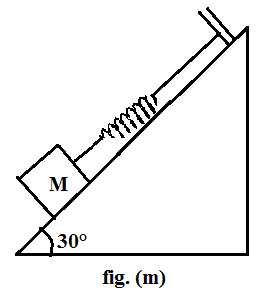(a) $\pi s$ (b) $\frac{\pi }{10}s$ (c) $\frac{2\pi }{5}s$ (d) $\frac{\pi }{2}s$

Solution:

$T=2\pi \sqrt{\frac{M}{K}}=2\pi \sqrt{\frac{0.1}{40}}$

= $\frac{\pi }{10}s$